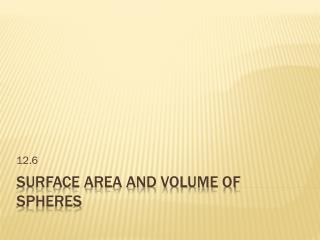DownloadDownload PresentationSurface Area and Volume of Spheres

# Surface Area and Volume of Spheres

Télécharger la présentation## Surface Area and Volume of Spheres

- - - - - - - - - - - - - - - - - - - - - - - - - - - E N D - - - - - - - - - - - - - - - - - - - - - - - - - - -
##### Presentation Transcript

1. 12.6 Surface Area and Volume of Spheres

2. Sphere – Set of all point in space equidistant from a given point. Center Radius – a segment from the center to a point on the sphere. Chord – A segment whose endpoints are on the sphere. Diameter – A chord that contain the center. Vocabulary

3. Vocab Pictures

4. The surface area S of a sphere is • S = 4 πr2 • Where r is the radius Surface Area

5. Find the surface area of the sphere. ANSWER The surface area of the sphere is about 804.25square inches. EXAMPLE 1 Find the surface area of a sphere SOLUTION S = 4πr2 Formula for surface area of a sphere. = 4π(82) Substitute 8 for r. = 256π Simplify. ≈ 804.25 Use a calculator.

6. ANSWER 2.71 for Examples 1 and 2 GUIDED PRACTICE 2. The surface area of a sphere is 30π square meters. Find the radius of the sphere.

7. The diameter of the outer sphere is 12feet, so the 12 radius is = 6feet. 2 EXAMPLE 3 Use the circumference of a sphere EXTREME SPORTS In a sport called sphereing, a person rolls down a hill inside an inflatable ball surrounded by another ball. The diameter of the outer ball is 12feet. Find the surface area of the outer ball. SOLUTION

8. ANSWER The surface area of the outer ball is 144π, or about 452.39 square feet. EXAMPLE 3 Use the circumference of a sphere Use the formula for the surface area of a sphere. S = 4πr2 = 4π(62) = 144π

9. Great Circle – a cross section of the sphere that contain the center Hemisphere – one half of the circle created by the great circle cross section. Vocab

10. Approximate the volume of the sphere as the sum of n pyramids each with a volume of V = (1/3)Bh. Volume

11. The diameter of the ball is 9 inches, so the radius is = 4.5 inches. 9 2 4 V = πr3 3 4 = π(4.5)3 3 EXAMPLE 4 Find the volume of a sphere The soccer ball has a diameter of 9 inches. Find its volume. SOLUTION Formula for volume of a sphere Substitute.

12. ANSWER The volume of the soccer ball is 121.5π, or about 381.70 cubic inches. Find the volume of a sphere EXAMPLE 4 = 121.5π Simplify. ≈ 381.70 Use a calculator.

13. Find the volume of the composite solid. 1 4 = πr2h – ( πr3) 2 3 2 = π(2)2(2) – π(2)3 3 2 = 8π – (8π) 3 Find the volume of a composite solid EXAMPLE 5 SOLUTION Formulas for volume Substitute. Multiply.

14. 24 16 = π – π 3 3 8 = π 3 ANSWER 8 The volume of the solid is π, or about 8.38 cubic inches. 3 Find the volume of a composite solid EXAMPLE 5 Rewrite fractions using least common denominator. Simplify.

15. 842-843 • 3-9 odd, 13,17,21-27 Homework